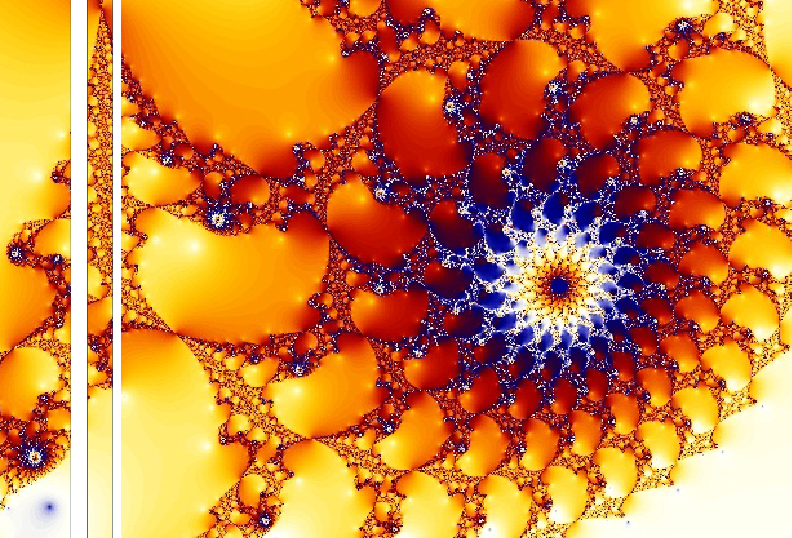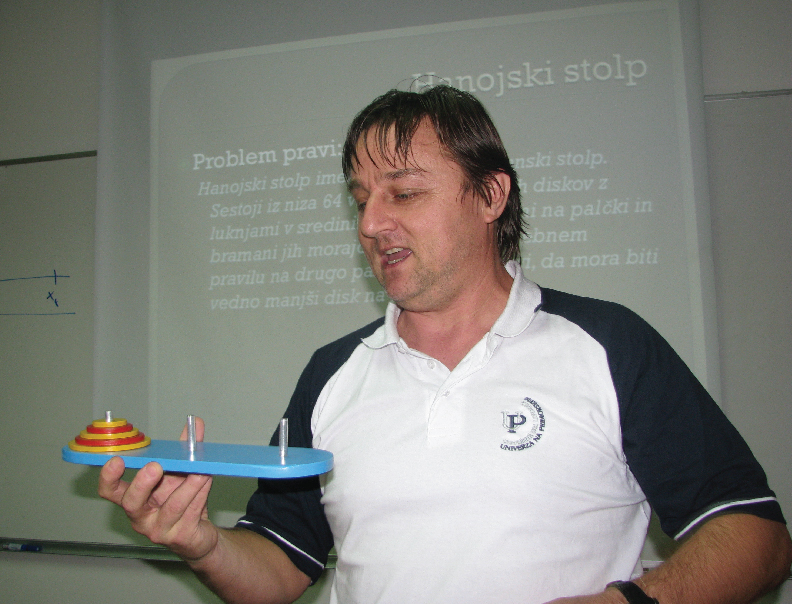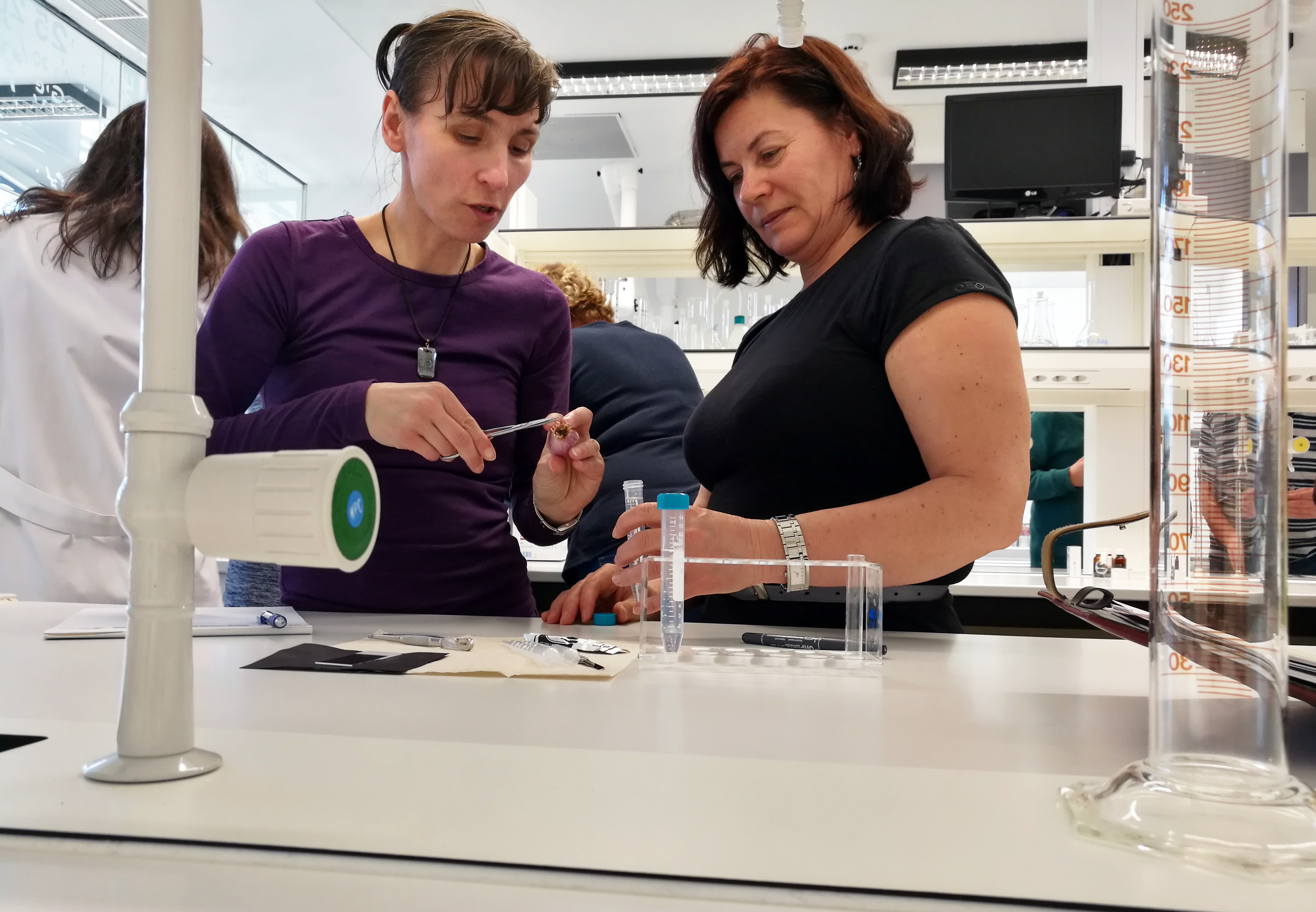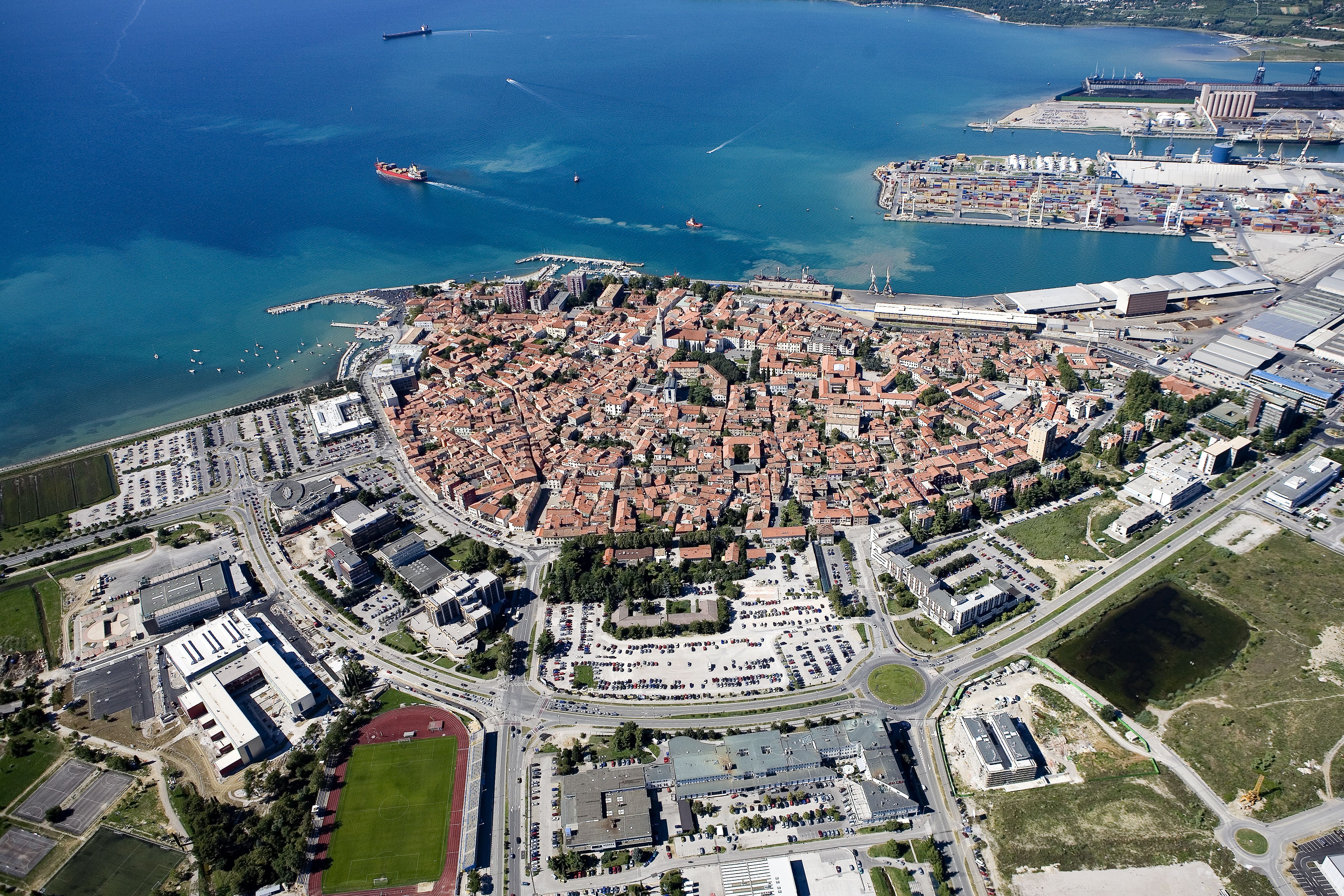Univerza na Primorskem Fakulteta za matematiko, naravoslovje in informacijske tehnologijeSI | EN# Raziskovalni matematični seminar - Arhiv

 2023 2022 2021 2020 2019 2018 2017 2016 2015 2014 2013 2012 2011 2010
 1 2 3 4 5 6 7 8 9 10 11 12
20.12.2010. ob 10:00 Seminarska soba v Galebu
Predavatelj: dr. Marko Orel

Naslov:  Adjacency preservers on symmetric matrices over a finite field

Povzetek: Let Sn(Fq) denote the set of all symmetric n×n matrices over a finite field Fq
with q elements. At the seminar the classification of all maps ϕ : Sn(Fq) →Sn(Fq),
which satisfy the implication:
if rk(A - B) = 1 then ”rk(ϕ (A) -ϕ (B))= 1;
will be presented. Here, rk(X) denotes the rank of a matrix X.
It turns out that any such map is necessarily bijective if n≥3. The results represents a generalization of the fundamental theorem of geometry of symmetric matrices (over a finite field). In the language of graph theory, the result says that the graph Gn with vertex set V (Gn) = Sn(Fq) and with edge set
E(Gn) = {{A;B}: rk(A - B) = 1} is a core, i.e., any its endomorphism is an automorphism, if n 3. This is not true if n = 2. In fact, it turns out that the chromatic number of G2 equals q and there exists a proper
endomorphism onto a clique of order q.